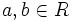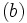# Dedekind-Hasse norm

## Statement

A Dedekind-Hasse norm on a commutative unital ring$R$ is a function$N$ from the nonzero elements of$R$ to the set of nonnegative integers, satisfying the following condition:

Whenever$a,b \in R$ are both nonzero, then one of these cases holds:

•$a$ is an element of the ideal$(b)$. In other words,$b | a$.
• There is a nonzero element in the ideal$(a,b)$ whose norm is strictly smaller than that of$b$.